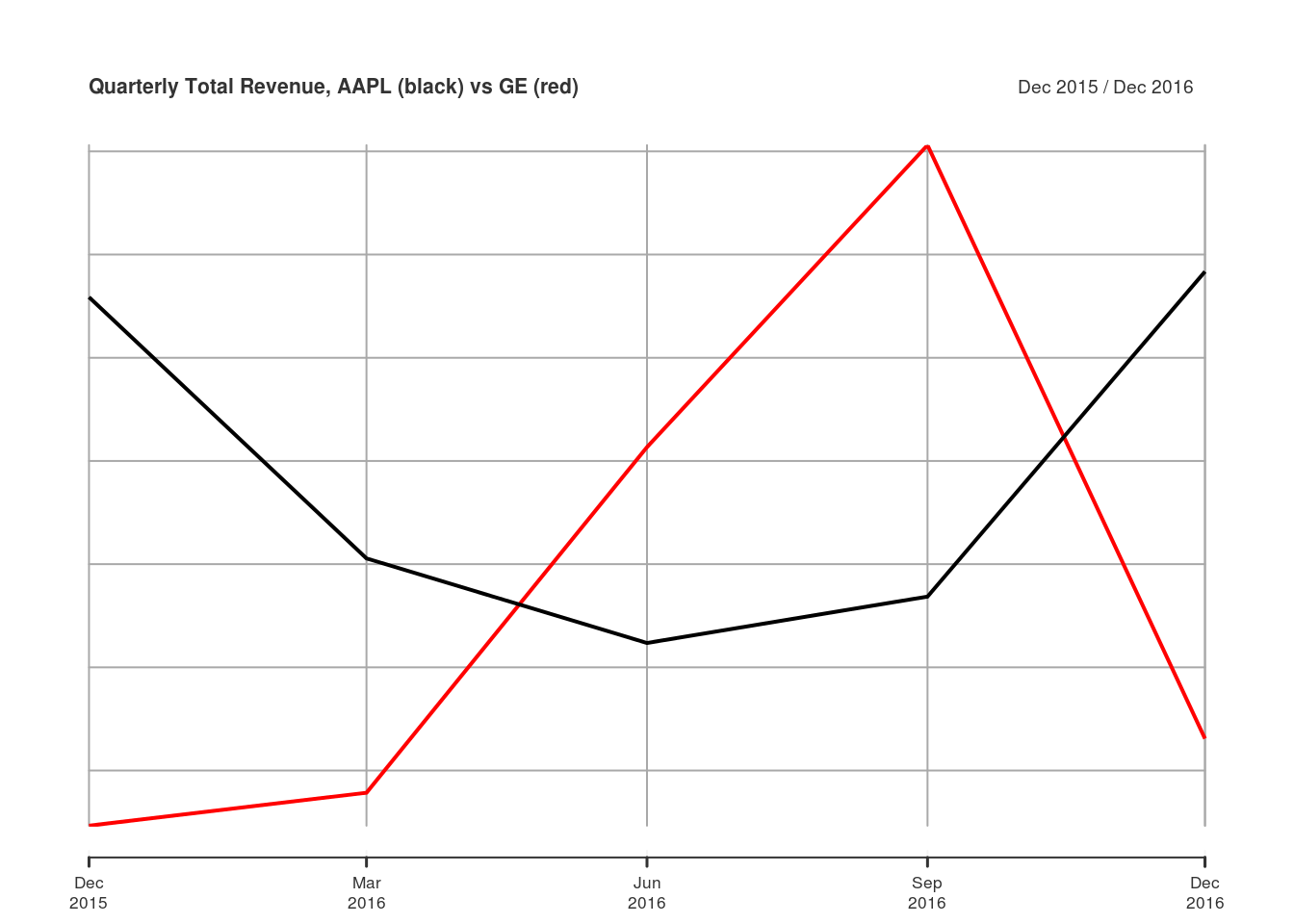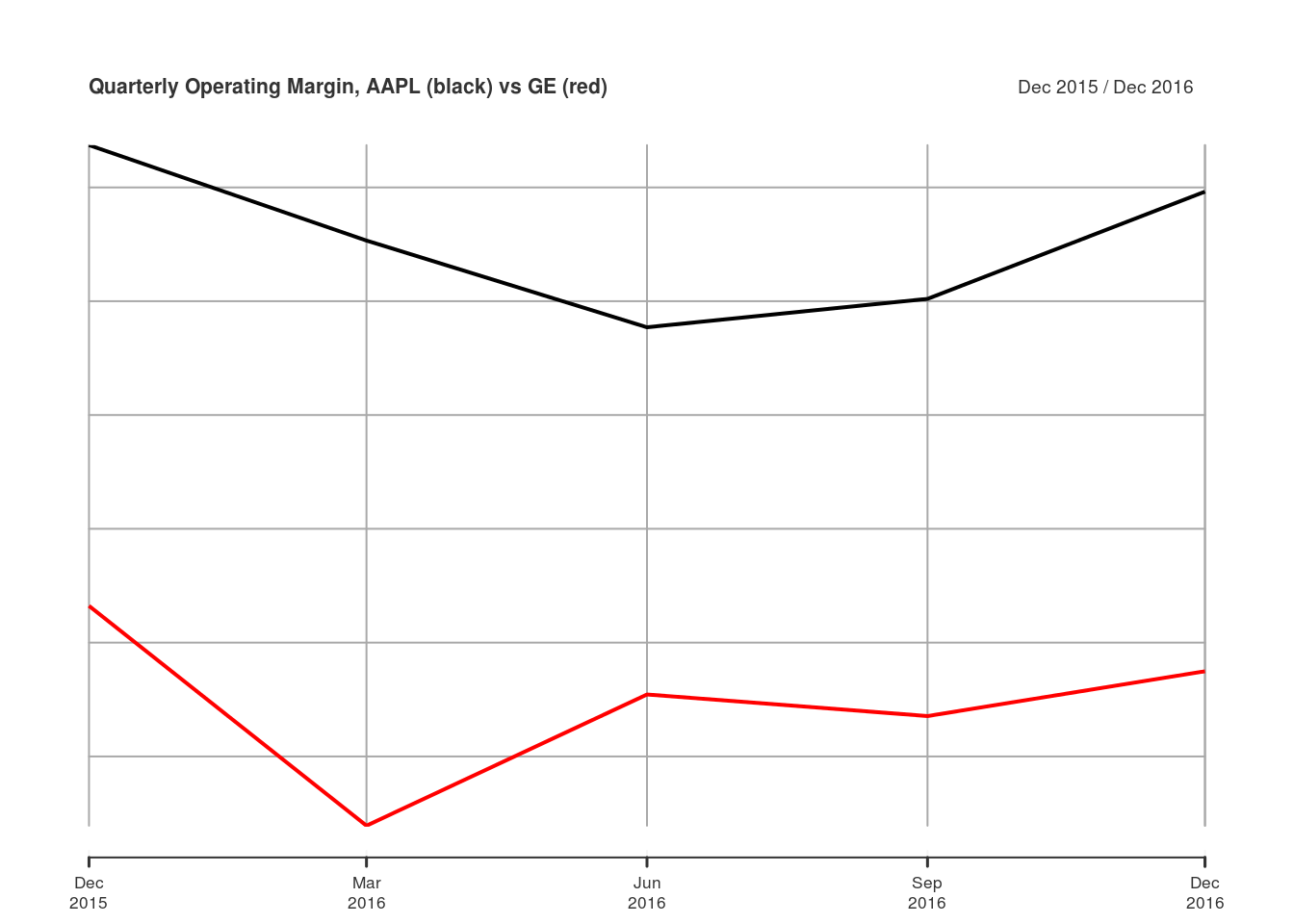# Stack Financials: Analyze Financial Statement Data

A quantmod user asked an interesting question on StackOverflow: Looping viewFinancials from quantmod. Basically, they wanted to create a `data.frame` that contained financial statement data for several companies for several years. I answered their question, and thought others might find the function I wrote useful… hence, this post!

I called the function `stackFinancials()` because it would use `getFinancials()` and `viewFinancials()` to pull financial statement data for multiple symbols, and stack them together in long form. I chose a long data format because I don’t know whether the output of `viewFinancials()` always has the same number of rows and columns for a given `type` and `period`. The long format makes it easy to put all the data in one object.

``````stackFinancials <-
function(symbols, type = c("BS", "IS", "CF"), period = c("A", "Q")) {
# Ensure the type and period arguments match viewFinancials
type <- match.arg(toupper(type), c("BS", "IS", "CF"))
period <- match.arg(toupper(period), c("A", "Q"))

# Simple function to get financials for one symbol
getOne <- function(symbol, type, period) {
gf <- getFinancials(symbol, auto.assign = FALSE)
vf <- viewFinancials(gf, type = type, period = period)
# Put viewFinancials output into a data.frame
df <- data.frame(vf, line.item = rownames(vf), type = type,
period = period, symbol = symbol,
stringsAsFactors = FALSE, check.names = FALSE)
# Reshape data.frame into long format
long <- reshape(df, direction="long", varying=seq(ncol(vf)),
v.names="value", idvar="line.item",
times=colnames(vf))
# Reset row.names to "automatic"
rownames(long) <- NULL
# Return data
long
}
# Loop over all symbols
allData <- lapply(symbols, getOne, type = type, period = period)
# rbind() all into one data.frame
do.call(rbind, allData)
}
``````

Here’s a simple example of how to use `stackFinancials()` to pull the quarterly (`period = "Q"`) income statements (`type = "IS"`) for General Electric and Apple:

``````library(quantmod)
Data <- stackFinancials(c("GE", "AAPL"), type = "IS", period = "Q")
##                line.item type period symbol       time value
## 1                Revenue   IS      Q     GE 2016-12-31 33088
## 2   Other Revenue, Total   IS      Q     GE 2016-12-31    NA
## 3          Total Revenue   IS      Q     GE 2016-12-31 33088
## 4 Cost of Revenue, Total   IS      Q     GE 2016-12-31 24775
``````

Now that we have the output in `Data`, let’s do something with it. You could simply subset `Data` to extract the components you want. For example, if you wanted to look at Apple’s quarterly revenue, you could subset `Data` where `symbol == "AAPL"` and `line.item == "Total Revenue"`. But if you’re going to slicing-and-dicing a lot, it can often help to write a general function to simplify things. So I wrote `extractLineItem()`. It takes the output of `stackFinancials()` and a regular expression of the line item you want, and it returns an xts object that contains the given line items for all symbols in the data.

``````extractLineItem <- function(stackedFinancials, line.item) {
if (missing(stackedFinancials) || missing(line.item)) {
stop("You must provide output from stackFinancials(),",
"and the line.item to extract")
}
# Select line items matching user input
match.rows <- grepl(line.item, Data\$line.item, ignore.case = TRUE)
sfSubset <- Data[match.rows,]
getItem <- function(x) {
# Create xts object
output <- xts(x\$value, as.yearmon(x\$time))
# Ensure column names are syntactically valid
valid.names <- make.names(paste(x\$symbol, x\$line.item))
# Remove repeating periods
colnames(output) <- gsub("\\.+", "\\.", valid.names)
output
}
# Split subset by line.item and symbol
symbol.item <- split(sfSubset, sfSubset[, c("symbol", "line.item")])
# Apply getItem() to each chunk, and merge into one object
do.call(merge, lapply(symbol.item, getItem))
}
``````

Let’s use `extractLineItem()` to compare total revenue for GE and AAPL.

``````totalRevenue <- extractLineItem(Data, "total revenue")
totalRevenue
##          AAPL.Total.Revenue GE.Total.Revenue
## Dec 2015              75872            24654
## Mar 2016              50557            27845
## Jun 2016              42358            61339
## Sep 2016              46852            90605
## Dec 2016              78351            33088
plot(totalRevenue, main = "Quarterly Total Revenue, AAPL (black) vs GE (red)")
``````You could also combine multiple calls to `extractLineItem()` to calculate ratios not included in the output from `viewFinancials()`. For example, you could divide operating income by total revenue to calculate operating margin.

``````operatingIncome <- extractLineItem(Data, "operating income")
operatingIncome
##          AAPL.Operating.Income GE.Operating.Income
## Dec 2015                 24171                2863
## Mar 2016                 13987                 545
## Jun 2016                 10105                4736
## Sep 2016                 11761                6138
## Dec 2016                 23359                2892
plot(operatingIncome / totalRevenue, main = "Quarterly Operating Margin, AAPL (black) vs GE (red)")
``````